Courses

# Types of Pie Charts CAT Notes | EduRev

## UPSC : Types of Pie Charts CAT Notes | EduRev

The document Types of Pie Charts CAT Notes | EduRev is a part of the UPSC Course UPSC CSAT: Logical Reasoning (LR) & Data Interpretation (DI).
All you need of UPSC at this link: UPSC

There are two types of pie charts:

(A) Normal Pie Chart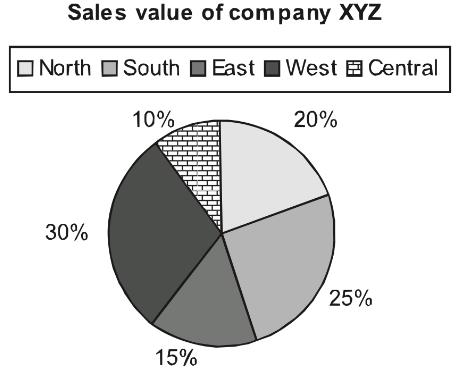This displays the contribution of each component of the pie.

(B) Exploded Pie Chart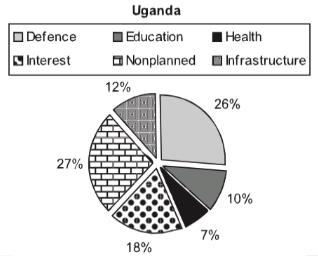(C) Mixed Layer Pie Chart

It is conventional to have multiple pie charts related to the same paragraph question to determine different aspects & drawing comparisons between the data. Once in CAT 2018 aspirants like you were posed with a multi-layered pie chart question like below; let’s solve the same pie chart to understand the concept better.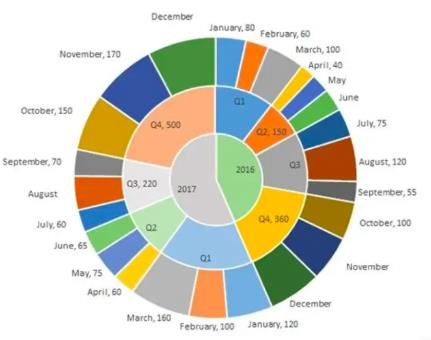The multi-layered pie-chart below shows the sales of LED television sets for a big retail electronics outlet during 2016 and 2017. The outer layer shows the monthly sales during this period, with each label showing the month followed by sales figure of that month. For some months, the sales figures are not given in the chart. The middle-layer shows quarter-wise aggregate sales figures (in some cases, aggregate quarter-wise sales numbers are not given next to the quarter). The innermost layer shows annual sales. It is known that the sales figures during the three months of the second quarter (April, May, June) of 2016 form an arithmetic progression, as do the three-monthly sales figures in the fourth quarter (October, November, December) of that year.

Q.1. What is the percentage increase in sales in December 2017 as compared to the sales in December 2016?
a.) 38.46
b.) 22.22
c.) 50.00
d.) 28.57
Q.2. In which quarter of 2017 was the percentage increase in sales from the same quarter of 2016 the highest?
a.) Q4
b.) Q1
c.) Q2
d.) Q3
Solution: To easily solve the questions we can first represent the information given in pie-chart in a consolidated table to make it easier to read and understand.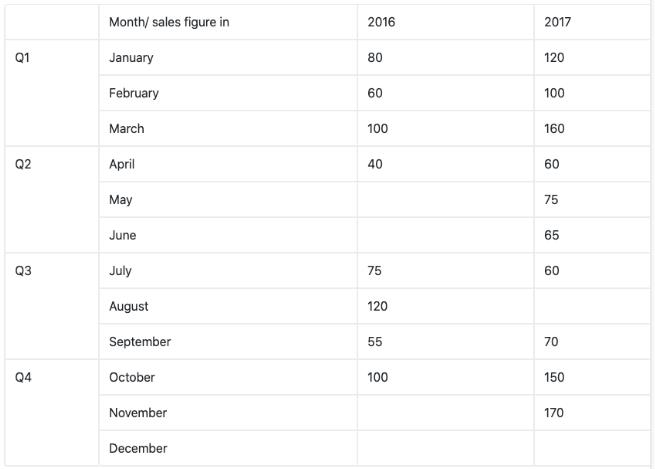As you can see in the above table there a few month’s sales are missing and we need to evaluate them first from the given information and then find the answers to above given question.

Now, it’s been given in the question that the months of April, May, June in 2016 forms an A.P. and so does the months of Quarter 4 of 2016, i.e. Oct, Nov, Dec.

Total sale in Quarter 2 = 150

Let a-d, a, a+d be the three terms of A.P.

Hence, a-d + a + a+d =150

• 3a = 150
• a = 50

Since, April sale is 40, i.e. a-d = 40

• d = 10

Using that we can easily find the rest of the month sales values i.e. 50 and 60 respectively.
Similarly, using the above method we can find out the values for the month of Nov’16 and Dec’16. In this case, a would-be 120 and d = 20.
Thus, sales for these months are 120 and 140 respectively.
Now as you can see in the table, we have found out all missing monthly sales figure except for December’17 and August’17 which can be easily find out as
Total sales in Quarter 4 of 2017 = 500
And sales in Oct’17 and Nov’17 are 150 and 170 respectively.
Hence, the sale for December = 500 -150-170
= 180
And similarly, Sale for August’17 = 220 – 60 – 70 = 90

Therefore, our table becomes,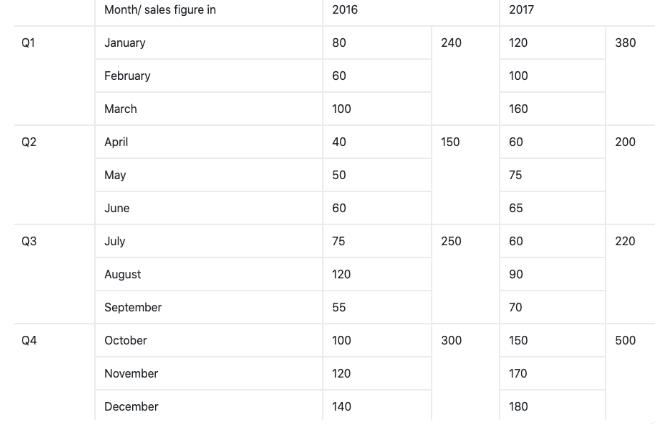Now we are in position to answer all the given questions using the above table.
Ans 1: Percentage increase in sales of Dec’17 as compared to Dec’16 =
Ans 2: We need to evaluate the quarter with the highest increase in sales in percentage terms.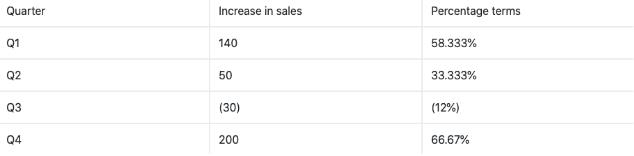Practice Questions:

Question 1:During which quarter was the percentage decrease in sales from the previous quarter’s sales the highest?

Question 2:During which month was the percentage increase in sales from the previous month’s sales the highest?

Offer running on EduRev: Apply code STAYHOME200 to get INR 200 off on our premium plan EduRev Infinity!

## UPSC CSAT: Logical Reasoning (LR) & Data Interpretation (DI)

76 videos|60 docs|106 tests

,

,

,

,

,

,

,

,

,

,

,

,

,

,

,

,

,

,

,

,

,

;